# Time Series Predictions with RNNs

Nikolaj Buhl
August 18, 2023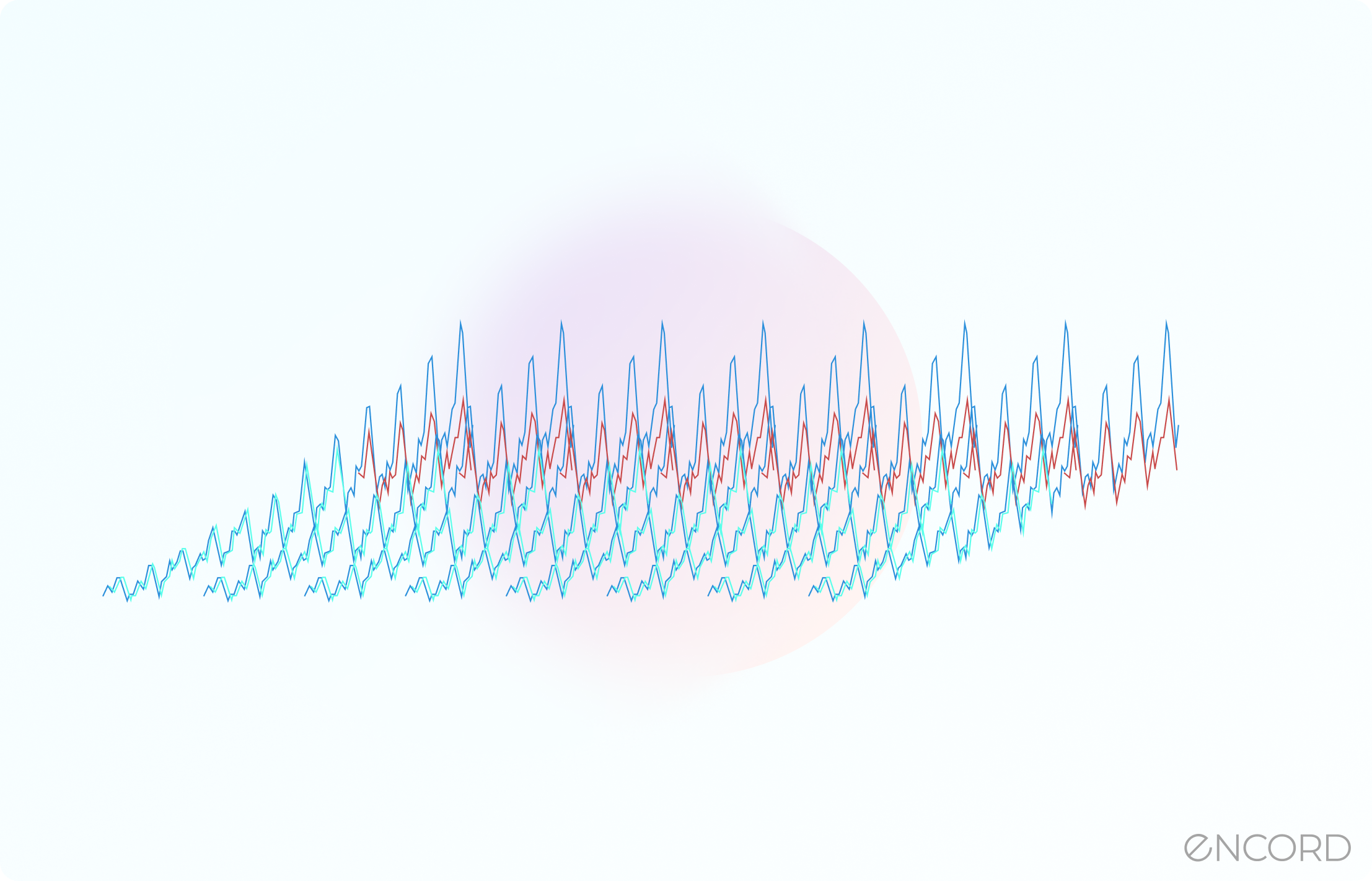Time series prediction, or time series forecasting, is a branch of data analysis and predictive modeling that aims to make predictions about future values based on historical data points in chronological order. In a time series, data is collected and recorded over regular intervals of time (i.e. hourly, daily, monthly, or yearly). Examples of time series data include stock prices, weather measurements, sales figures, website traffic, and more.

Recurrent Neural Networks (RNNs) are deep learning models that can be utilized for time series analysis, with recurrent connections that allow them to retain information from previous time steps. Popular variants include Long Short-Term Memory (LSTM) and Gated Recurrent Unit (GRU), which can learn long-term dependencies.

• Time Series Data
• Recurrent Neural Networks (RNNs)
• Building and training the Recurrent Neural Networks (RNN) Model
• Evaluating the Model Performance
• Limitations of Time Series Predictions with Recurrent Neural Networks (RNNs)
• Advanced Techniques for Time Series Predictions
• Applying Recurrent Neural Networks (RNNs) on real data (Using Python and Keras)To learn about activation functions, read: Activation Functions in Neural Networks: With 15 examples.

Build Better Models, Faster with Encord's Leading Annotation Tool

## What is Time Series Data?

Time series data is a sequence of observations recorded over time, where each data point is associated with a specific timestamp. This data type is widely used in various fields to analyze trends, make predictions, and understand temporal patterns. Time series data has unique characteristics, such as temporal ordering, autocorrelation (where a data point depends on its previous ones), and seasonality (repeating patterns over fixed intervals).Recurrent Neural Networks (RNNs) bring a unique edge to time series forecasting, empowering you to capture intricate temporal dependencies. Exploring the realm of Long Short-Term Memory (LSTM) and Gated Recurrent Unit (GRU) models unveils the genuine potential of predictive analytics.

### Types of Time Series Patterns

Time series data analysis involves identifying various patterns that provide insights into the underlying dynamics of the data over time. These patterns shed light on the trends, fluctuations, and noise present in the dataset, enabling you to make informed decisions and predictions. Let's explore some of the prominent time series patterns that help us decipher the intricate relationships within the data and leverage them for predictive analytics.

From discerning trends and seasonality to identifying cyclic patterns and understanding the impact of noise, each pattern contributes to our understanding of the data's behavior over time. Additionally, time series regression introduces a predictive dimension, allowing you to forecast numerical values based on historical data and the influence of other variables.

Delving into the below patterns not only offers a world of insights within time-dependent data but also unearths distinct components that shape its narrative:

• Trends: Trends represent long-term changes or movements in the data over time. These can be upward (increasing trend) or downward (decreasing trend), indicating the overall direction in which the data is moving.
• Seasonality: Seasonality refers to repeating patterns or fluctuations that occur at regular intervals. These patterns might be daily, weekly, monthly, or yearly, depending on the nature of the data.
• Cyclic Patterns: Unlike seasonality, cyclic patterns are not fixed to specific intervals and may not repeat at regular frequencies. They represent oscillations that are not tied to a particular season.
• Noise: Noise is the random variation present in the data which does not follow any specific pattern. It introduces randomness and uncertainty to the time series.
• Regression: Time series regression involves building a predictive model to forecast a continuous numerical value (the dependent variable) based on historical time series data of one or more predictors (independent variables).

### Preprocessing Techniques for Time Series Data

Before applying any prediction model, proper preprocessing is essential for time series data. Some common preprocessing techniques include:

• Handling Missing Values: Addressing missing values is crucial as gaps in the data can affect the model's performance. You can use techniques like interpolation or forward/backward filling.
• Data Normalization: Normalizing the data ensures that all features are on the same scale, preventing any single feature from dominating the model's learning process.
• Detrending: Removing the trend component from the data can help in better understanding the underlying patterns and making accurate predictions.
• Seasonal Adjustment: For data with seasonality, seasonal adjustment methods like seasonal differencing or seasonal decomposition can be applied.
• Smoothing: Smoothing techniques like moving averages can be used to reduce noise and highlight underlying patterns.
• Train-test Split: It is crucial to split the data into training and test sets while ensuring that the temporal order is maintained. This allows the model to learn from past input data of the training set and evaluate its performance on unseen future data.Mastering the train-validation-test split is crucial for robust machine learning models. Learn how to segment datasets effectively, prevent overfitting, and optimize model performance in our comprehensive guide on Training, Validation, and Test Split for Machine Learning Datasets.

With a grasp of the characteristics of time series data and the application of suitable preprocessing methods, you can lay the groundwork for constructing resilient predictive models utilizing Recurrent Neural Networks (RNNs).

## Recurrent Neural Networks (RNNs)

A Recurrent Neural Network (RNN) is like a specialized brain for handling sequences, such as sentences or time-based data. Imagine it as a smart cell with its own memory. For example, think about predicting words in a sentence. The RNN not only understands each word but also remembers what came before using its internal memory. This memory helps it capture patterns and relationships in sequences. This makes RNNs great for tasks like predicting future values in time series data, like stock prices or weather conditions, where past information plays a vital role.

Recurrent Neural Networks (RNNs) offer several advantages for time series prediction tasks. They can handle sequential data of varying lengths, capturing long-term dependencies and temporal patterns effectively. RNNs accommodate irregularly spaced time intervals and adapt to different forecasting tasks with input and output sequences of varying lengths.

However, RNNs have limitations like the vanishing or exploding gradient problem, which affects their ability to capture long-term dependencies because RNNs may be unrolled very far back in this Memory constraints may also limit their performance with very long sequences. While techniques like LSTMs and GRUs mitigate some issues, other advanced architectures like Transformers might outperform RNNs in certain complex time series scenarios, necessitating careful model selection.

### Limitations

The vanishing gradient problem is a challenge that affects the training of deep neural networks, including Recurrent Neural Networks (RNNs). It occurs when gradients, which indicate the direction and magnitude of updates to network weights during training, become very small as they propagate backward through layers. This phenomenon hinders the ability of RNNs to learn long-range dependencies and can lead to slow or ineffective training.

The vanishing gradient problem is particularly problematic in sequences where information needs to be remembered or propagated over a long span of time, affecting the network's ability to capture important patterns. To combat the vanishing gradient problem that hampers effective training in neural networks, several strategies have emerged. Techniques like proper weight initialization, batch normalization, gradient clipping, skip connections, and learning rate scheduling play pivotal roles in stabilizing gradient flow and preventing their untimely demise.

Amid this spectrum of approaches, two standout solutions take center stage: LSTM (Long Short-Term Memory) and GRU (Gated Recurrent Unit).

### Long Short-Term Memory (LSTM) and Gated Recurrent Unit (GRU)

Traditional RNNs struggle with the vanishing gradient problem, which makes it difficult for the network to identify long-term dependencies in sequential data. However, this challenge is elegantly addressed by LSTM, as it incorporates specialized memory cells and gating mechanisms that preserve and control the flow of gradients over extended sequences. This enables the network to capture long-term dependencies more effectively and significantly enhances its ability to learn from sequential data. LSTM has three gates (input, forget, and output) and excels at capturing long-term dependencies.

Gated Recurrent Unit (GRU), a simplified version of LSTM with two gates (reset and update), maintains efficiency and performance similar to LSTM, making it widely used in time series tasks.In 1997, Sepp Hochreiter and Jürgen Schmidhuber published a seminal paper titled "Long Short-Term Memory" that introduced the LSTM network. Both the scientists are pioneers in the field of Artificial Intelligence and Machine Learning.

## Building and Training the Recurrent Neural Networks (RNNs) Model for Time Series Predictions

Building and training an effective RNN model for time series predictions requires an approach that balances model architecture and training techniques. This section explores all the essential steps for building and training an RNN model. The process includes data preparation, defining the model architecture, building the model, fine-tuning hyperparameters, and then evaluating the model’s performance.

### Data Preparation

Data preparation is crucial for accurate time series predictions with RNNs. Handling missing values and outliers, scaling data, and creating appropriate input-output pairs are essential. Seasonality and trend removal help uncover patterns, while selecting the right sequence length balances short- and long-term dependencies.

Feature engineering, like lag features, improves model performance. Proper data preprocessing ensures RNNs learn meaningful patterns and make accurate forecasts on unseen data.

### Building the RNN Model

Building the RNN model includes a series of pivotal steps that collectively contribute to the model’s performance and accuracy.

• Designing the RNN Architecture: Constructing the RNN architecture involves deciding the layers and the neurons in the network. A typical structure for time series prediction comprises an input layer, one or more hidden layers with LSTM or GRU cells and an output layer.
• Selecting the optimal number of layers and neurons: This is a critical step in building the RNN model. Too few layers or neurons may lead to underfitting, while too many can lead to overfitting. It's essential to strike a balance between model complexity and generalization. You can use techniques like cross-validation and grid search to find the optimal hyperparameters.
• Hyperparameter tuning and optimization techniques: Hyperparameter tuning involves finding the best set of hyperparameters for the RNN model. Hyperparameters include learning rate, batch size, number of epochs, and regularization strength. You can employ grid or randomized search to explore different combinations of hyperparameters and identify the configuration that yields the best performance.

Hyperparameter Tuning

### Training the Recurrent Neural Networks (RNNs) Model

Training an RNN involves presenting sequential data with learning algorithms to the model and updating its parameters iteratively to minimize the prediction error.

By feeding historical sequences into the RNN, it learns to capture patterns and dependencies in the data. The process usually involves forward propagation to compute predictions and backward propagation to update the model's weights using optimization algorithms like Stochastic Gradient Descent (SGD) or Adam.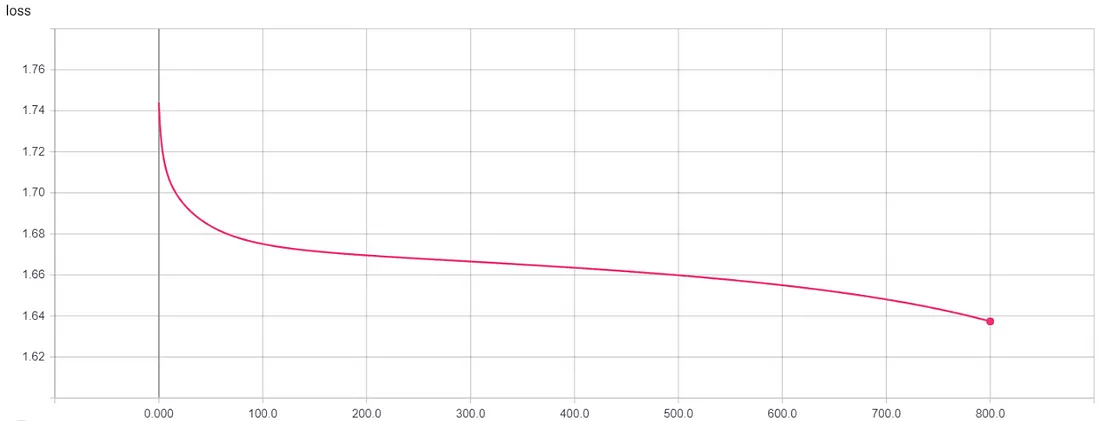Stochastic Gradient Descent, Learning Rate = 0.01

Backpropagation through time (BPTT) is a variant of the standard backpropagation algorithm used in RNNs.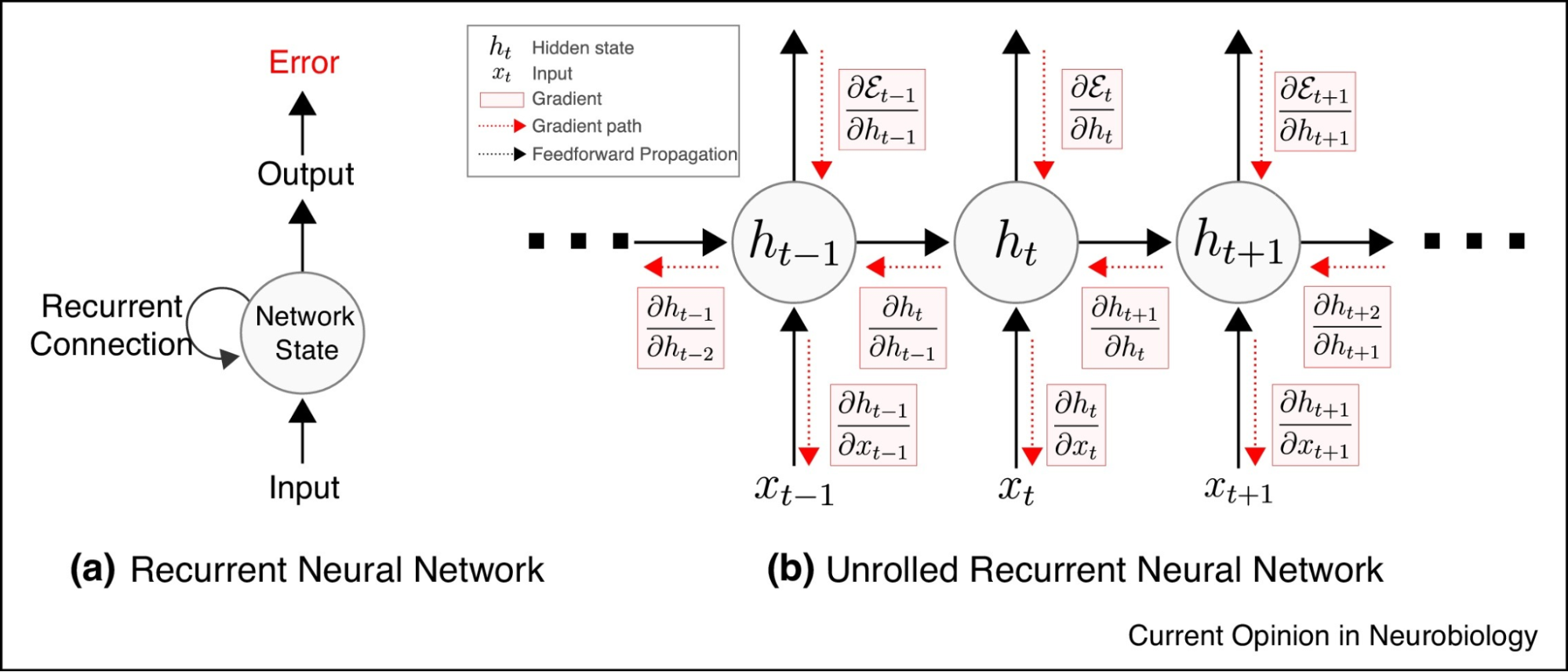Backpropagation through time (BPTT)

Overfitting is a common issue in deep learning models, including RNNs. You can employ regularization techniques like L1 and L2 regularization, dropout, and early stopping to prevent overfitting and improve the model's generalization performance.

## Evaluating Model Performance

To assess the performance of the trained RNN model, you can use evaluation metrics such as Mean Absolute Error (MAE), Root Mean Squared Error (RMSE), and Mean Absolute Percentage Error (MAPE). These metrics quantify the accuracy of the predictions compared to the actual values and provide valuable insights into the model's effectiveness.

Visualizing the model's predictions against the actual time series data can help you understand its strengths and weaknesses. Plotting the predicted values alongside the true values provides an intuitive way to identify patterns, trends, and discrepancies. Interpreting the results involves analyzing the evaluation metrics, visualizations, and any patterns or trends observed.

Based on the analysis, you can identify potential improvements to the model. These may include further tuning hyperparameters, adjusting the architecture, or exploring different preprocessing techniques. By carefully building, training, and evaluating the RNN model, you  can develop a powerful tool for time series prediction that can capture temporal dependencies and make accurate forecasts.

## Limitations of Time Series Predictions with Recurrent Neural Networks (RNNs)

While Recurrent Neural Networks (RNNs) offer powerful tools for time series predictions, they have certain limitations. Understanding these limitations is crucial for developing accurate and reliable predictive models. RNNs may struggle with capturing long-term dependencies, leading to potential prediction inaccuracies.

Additionally, training deep RNNs can be computationally intensive, posing challenges for real-time applications. Addressing these limitations through advanced architectures and techniques is essential to harnessing the full potential of RNNs in time series forecasting.

### Non-Stationary Time Series Data

Non-stationary time series data exhibits changing statistical properties such as varying mean or variance, over time. Dealing with non-stationarity is crucial, as traditional models assume stationarity.

Techniques like differencing, detrending, or seasonal decomposition can help transform the data into a stationary form. Additionally, advanced methods like Seasonal Autoregressive Integrated Moving Average (SARIMA) or Prophet can be used to model and forecast non-stationary time series.

### Data with Irregular Frequencies and Missing Timestamps

Real-world time series data can have irregular frequencies and missing timestamps, disrupting the model's ability to learn patterns. You can apply resampling techniques (e.g., interpolation, aggregation) to convert data to a regular frequency. For missing timestamps, apply imputation techniques like forward and backward filling or more advanced methods like time series imputation models.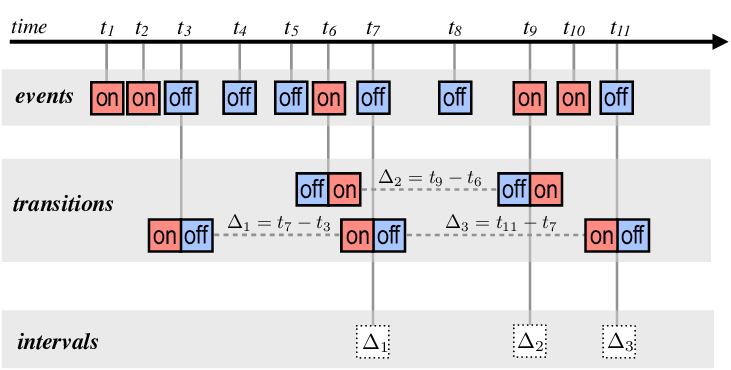Time series data: a single pixel produces an irregular series of raw events

### Concept Drift and Model Adaptation

In dynamic environments, time series data might undergo concept drift, where the underlying patterns and relationships change over time. To address this, the model needs to adapt continuously. Use techniques like online learning and concept drift detection algorithms to monitor data distribution changes and trigger model updates when necessary.

As time series data becomes more complex and diverse, advanced techniques are essential to enhance the capabilities of Recurrent Neural Networks (RNNs). Multi-variate time series data featuring multiple interconnected variables can be effectively handled by extending RNNs to accommodate multiple input features and output predictions. Incorporating attention mechanisms refines RNN predictions by prioritizing relevant time steps or features, especially in longer sequences.

Also, combining RNNs with other models like CNN-RNN, Transformer-RNN, or ANN-RNN makes hybrid architectures that can handle both spatial and sequential patterns. This improves the accuracy of predictions in many different domains. These sophisticated techniques empower RNNs to tackle intricate challenges and deliver comprehensive insights.

### Multi-Variate Time Series Data in Recurrent Neural Networks (RNNs)

In many real-world scenarios, time series data may involve multiple related variables. You can extend RNNs to handle multi-variate time series by incorporating multiple input features and predicting multiple output variables. This allows the model to leverage additional information to make more accurate predictions and better capture complex relationships among different variables.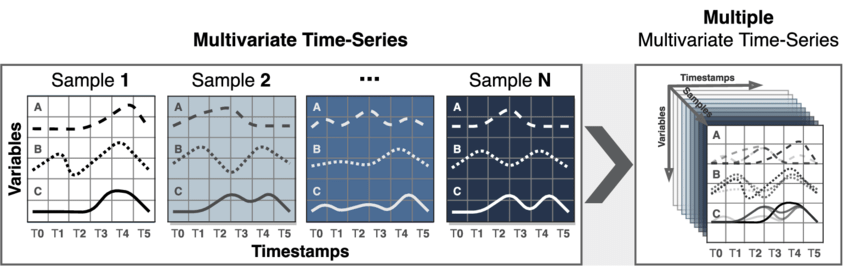Multivariate Time Series

### Attention Mechanisms for More Accurate Predictions

Attention mechanisms enhance RNNs by focusing on relevant time steps or features during predictions. They improve accuracy and interpretability, especially in long sequences. Combining RNNs with other models, like the convolutional neural network model CNN-RNN or Transformer-RNN, Artificial Neural Networks ANN-RNN, may further boost performance for time series tasks.

ANNs consist of interconnected artificial neurons, nodes or units, organized into layers. Hybrid models effectively handle spatial and sequential patterns, leading to better domain predictions and insights. Advanced techniques like Seq-2-Seq, bidirectional, transformers etc. make RNNs more adaptable, addressing real-world challenges and yielding comprehensive results.

## Case Study: Applying Recurrent Neural Networks (RNNs) to Real Data

This case study uses Recurrent Neural Networks (RNNs) to predict electricity consumption based on historical data. The "Electricity Consumption'' dataset contains hourly electricity consumption data over a period of time. The aim is to build an RNN model to forecast future electricity consumption, leveraging past consumption patterns.

Python Code (using Pytorch):

import numpy as np
import pandas as pd
import matplotlib.pyplot as plt
from sklearn.preprocessing import MinMaxScaler
import torch
import torch.nn as nn
from sklearn.metrics import mean_absolute_error, mean_absolute_percentage_error, mean_squared_error

# Sample data for Electricity Consumption
data = {
'timestamp': pd.date_range(start='2023-01-01', periods=100, freq='H'),
'consumption': np.random.randint(100, 1000, 100)
}
df = pd.DataFrame(data)
df.set_index('timestamp', inplace=True)

# Preprocessing
scaler = MinMaxScaler()
df_scaled = scaler.fit_transform(df)

# Create sequences and labels for training
seq_length = 24
X, y = [], []
for i in range(len(df_scaled) - seq_length):
X.append(df_scaled[i:i + seq_length])
y.append(df_scaled[i + seq_length])

X, y = np.array(X), np.array(y)

# Split the data into training and test sets
train_size = int(0.8 * len(X))
X_train, X_test = X[:train_size], X[train_size:]
y_train, y_test = y[:train_size], y[train_size:]

# Create a custom dataset class for PyTorch DataLoader
class TimeSeriesDataset(Dataset):
def __init__(self, X, y):
self.X = torch.tensor(X, dtype=torch.float32)
self.y = torch.tensor(y, dtype=torch.float32)

def __len__(self):
return len(self.X)

def __getitem__(self, index):
return self.X[index], self.y[index]

# Define the RNN model
class RNNModel(nn.Module):
def __init__(self, input_size, hidden_size, output_size):
super(RNNModel, self).__init__()
self.rnn = nn.LSTM(input_size, hidden_size, batch_first=True)
self.fc = nn.Linear(hidden_size, output_size)

def forward(self, x):
out, _ = self.rnn(x)
out = self.fc(out[:, -1, :])
return out

# Hyperparameters
input_size = X_train.shape
hidden_size = 128
output_size = 1
learning_rate = 0.001
num_epochs = 50
batch_size = 64

train_dataset = TimeSeriesDataset(X_train, y_train)

# Initialize the model, loss function, and optimizer
model = RNNModel(input_size, hidden_size, output_size)
criterion = nn.MSELoss()

# Training the model
for epoch in range(num_epochs):
outputs = model(inputs)
loss = criterion(outputs, targets)
loss.backward()
optimizer.step()

if (epoch + 1) % 10 == 0:
print(f'Epoch [{epoch+1}/{num_epochs}], Loss: {loss.item():.4f}')

# Evaluation on the test set
model.eval()
X_test_tensor = torch.tensor(X_test, dtype=torch.float32)
y_pred = model(X_test_tensor).numpy()
y_pred = scaler.inverse_transform(y_pred)
y_test = scaler.inverse_transform(y_test)

# Calculate RMSE
mse = mean_squared_error(y_test, y_pred)
rmse = np.sqrt(mse)
print(f"Root Mean Squared Error (RMSE): {rmse}")
mae = mean_absolute_error(y_test, y_pred)
print(f"Mean Absolute Error (MAE): {mae:.2f}")
mape = mean_absolute_percentage_error(y_test, y_pred) * 100
print(f"Mean Absolute Percentage Error (MAPE): {mape:.2f}%")

# Visualize predictions against actual data
plt.figure(figsize=(10, 6))
plt.plot(df.index[train_size+seq_length:], y_test, label='Actual')
plt.plot(df.index[train_size+seq_length:], y_pred, label='Predicted')
plt.xlabel('Timestamp')
plt.ylabel('Electricity Consumption')
plt.title('Electricity Consumption Prediction using RNN (PyTorch)')
plt.legend()
plt.show()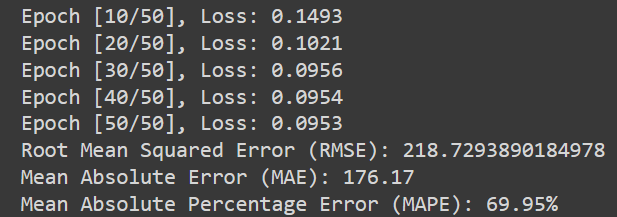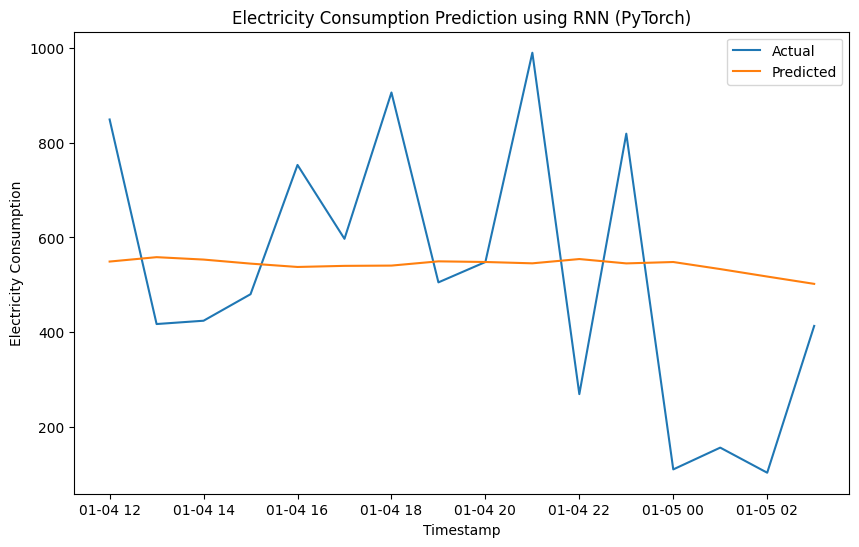Time Series with Recurrent Neural Networks RNN - Github

The provided code demonstrates the implementation of a Recurrent Neural Network (RNN) using PyTorch for electricity consumption prediction. The model is trained and evaluated on a sample dataset. The training process includes 50 epochs, and the loss decreases over iterations, indicating the learning process.

The analysis reveals significant gaps between predicted and actual electricity consumption values, as indicated by the relatively high Root Mean Squared Error (RMSE), Mean Absolute Error (MAE), and Mean Absolute Percentage Error (MAPE). These metrics collectively suggest that the current model's predictive accuracy requires improvement. The deviations underscore that the model falls short in capturing the true consumption patterns accurately.

In the context of the case study, where the goal is to predict electricity consumption using Recurrent Neural Networks (RNNs), these results highlight the need for further fine-tuning.

Despite leveraging historical consumption data and the power of RNNs, the model's performance indicates a discrepancy between predicted and actual values. The implication is that additional adjustments to the model architecture, hyperparameters, or preprocessing of the dataset are crucial. Enhancing these aspects could yield more reliable predictions, ultimately leading to a more effective tool for forecasting future electricity consumption patterns."RNNs have revolutionized time series analysis, enabling us to predict future values with remarkable accuracy. Through the lens of LSTM and GRU, you can decipher hidden patterns within temporal data, paving the way for transformative insights in diverse industries." - Dr. John Smith

## Time Series Predictions with Recurrent Neural Networks (RNNs): Key Takeaways

• Time series data possesses unique characteristics, necessitating specialized techniques for analysis and forecasting.
• Recurrent Neural Networks (RNNs) excel in handling sequences, capturing dependencies, and adapting to diverse tasks.
• Proper data preparation, model building, and hyperparameter tuning are crucial for successful RNN implementation.
• Evaluation metrics and visualization aid in assessing model performance and guiding improvements.
• Addressing real-world challenges requires advanced techniques like attention mechanisms and hybrid models.

## Time Series Predictions with Recurrent Neural Networks (RNNs): Frequently Asked Questions

### What is time series data?

Time series data is a sequence of observations recorded over time, often used in fields like finance and weather forecasting. Its uniqueness lies in temporal ordering, autocorrelation, seasonality, cyclic patterns, and noise, which necessitate specialized techniques for analysis and prediction.

### What are recurrent neural networks (RNNs) and their advantages?

RNNs are specialized neural networks designed for sequential data analysis. They excel in handling varying sequence lengths, capturing long-term dependencies, and adapting to irregular time intervals. RNNs are proficient in tasks requiring an understanding of temporal relationships.

### How do LSTM and GRU models address challenges like the vanishing gradient problem?

Long Short-Term Memory (LSTM) and Gated Recurrent Unit (GRU) models are RNN variations that mitigate the vanishing gradient problem. They incorporate gating mechanisms that allow them to retain information from previous time steps, enabling the learning of long-term dependencies.

### What challenges do recurrent neural networks (RNNs) face, and how can they be overcome?

While RNNs offer powerful capabilities, they also have limitations, including computational demands and potential struggles with very long sequences. Addressing these challenges requires meticulous hyperparameter tuning, careful data preparation, and techniques like regularization.

### How can recurrent neural networks (RNNs) be applied to real-world time series data?

Applying RNNs to real-world time series data involves a comprehensive process. It begins with proper data preprocessing, designing the RNN architecture, tuning hyperparameters, and training the model. Evaluation metrics and visualization are used to assess performance and guide improvements, addressing challenges like non-stationarity, missing timestamps, and more.

Scale your annotation workflows and power your model performance with data-driven insights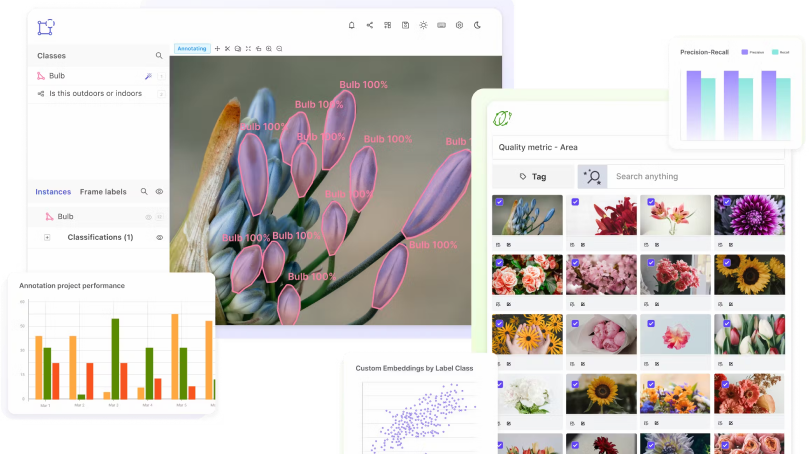Written by Nikolaj Buhl
Nikolaj is a Product Manager at Encord and a computer vision enthusiast. At Encord he oversees the development of Encord Active. Nikolaj holds a M.Sc. in Management from London Business School and Copenhagen Business School. In a previous life, he lived in China working at the Danish Embas... see more#### Discuss this blog on Slack

Join the Encord Developers community to discuss the latest in computer vision, machine learning, and data-centric AI

Join the community
###### Subscribe